Using the Excel SUMIF Function: Everything You Need to Know

Izabelle Hundrev  |  September 26, 2019

Microsoft Excel has many capabilities.

One of the most useful features are the preset Excel formulas, also referred to as functions. Sometimes working with data requires you to pull certain values and not others. If you’re looking to add a range of values that need to meet a certain criterion, the SUMIF function is going to be your best friend.

In this article, we’ll cover the SUMIF equation, its arguments, and how to use the SUMIF function in a real example.

How to use SUMIF in Excel

Using SUMIF in Excel is fairly simple if you know how and when to use the function. As mentioned before, SUMIF is for adding values that meet a specific criteria. For example, let’s say you want to find the sum of all values greater than 5 in your worksheet. This is a situation when you would use SUMIF.

 TIP: Just looking to do simple addition? No need to use the SUMIF function. Instead, learn how to add in Excel.

First, let’s break down the nuts and bolts.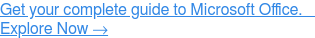The syntax

In Excel, syntax refers to the arguments that make up the function. The syntax formula for SUMIF is as follows:

=SUMIF(range, criteria, [sum_range])

Don’t forget the equals sign (=), every Excel formula begins with the same symbol.

The arguments

For the formula to work correctly, you need to know the proper inputs, also referred to as arguments. We’ve compiled some quick definitions of each argument in the SUMIF formula below:

Range

The range is the array of cells evaluated by your criteria. This is a required input.

Criteria

The criteria is the condition that needs to be met. This can be a number, text, or an expression. For example, “Thursday”, “<10,” and “oranges” are all acceptable inputs. This is also a required input.

Sum range

This input is optional. The sum range is the range of values, or cells, that will be added together if the range values meet the specified criteria. If this input is omitted, Excel will pull the sum of the range argument instead.

 Related: Do these arguments look familiar? You're likely thinking of the Excel COUNTIF function, which is very similar but has a slightly different use case.

Using SUMIF in an example

Let’s see SUMIF in action. In this example, we have a list of vegetables, the state that supplies them, and the quantity in pounds.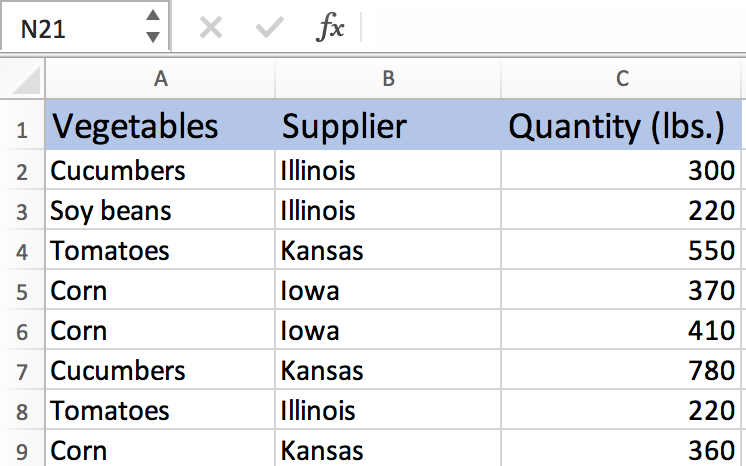Let’s say we want to find out the total quantity of vegetables supplied by Illinois.

Step 1: In the next column, type in “Supplier” and “Quantity”

Since we want to find out the quantity of vegetables for Illinois, type in “Illinois” in the open cell next to “Supplier.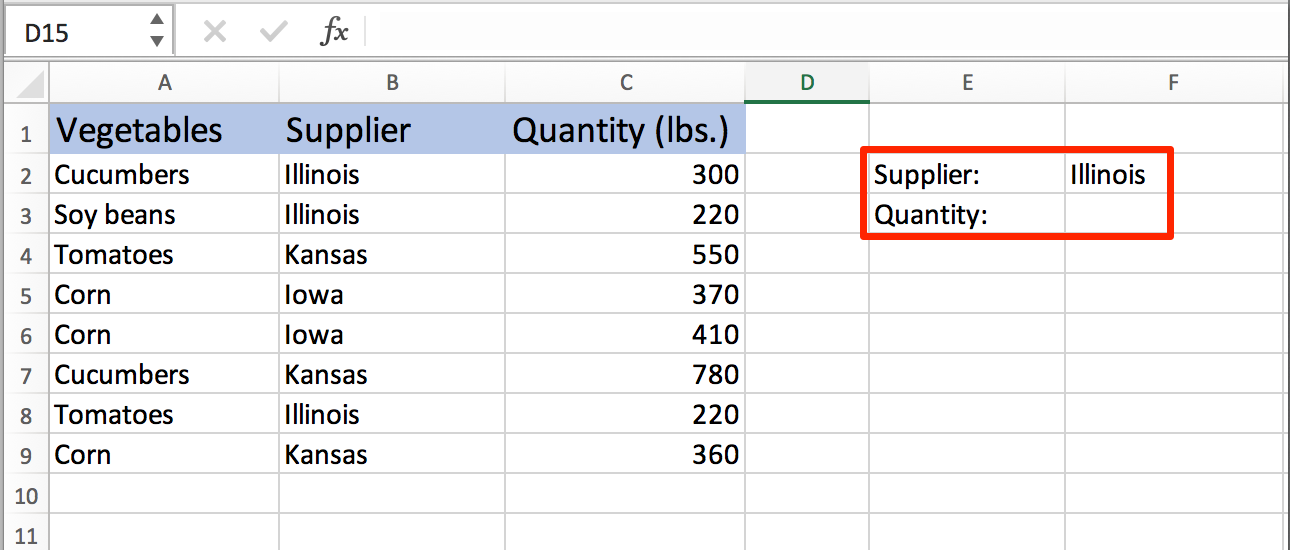Step 2: Enter the SUMIF formula in the cell next to “Quantity”

Select the cell next to “Quantity.” Here you will enter the SUMIF formula. Begin by typing out an equals sign (=), followed by selecting SUMIF when the name pops up. Excel will automatically pull the formula for you.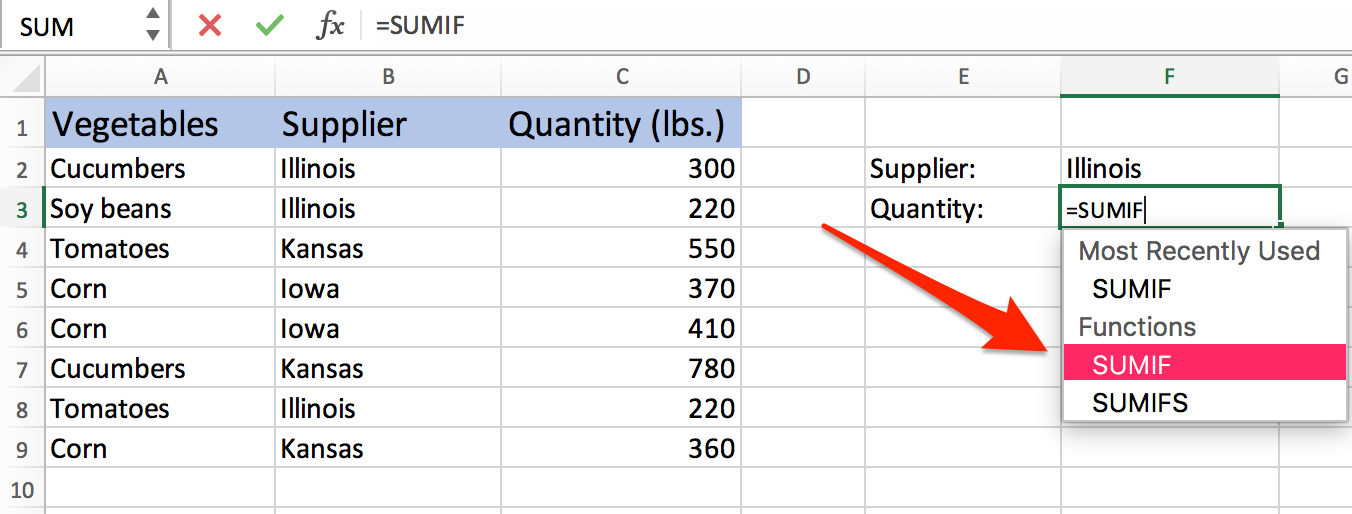Step 3: Fill in the arguments

Once Excel pulls the formula, it’s necessary to define which range of cells fits in where.

In this example, the arguments are defined as:

• Range: B2:B9
• Criteria: F2
• Sum range: C2:C9

See the image below for reference: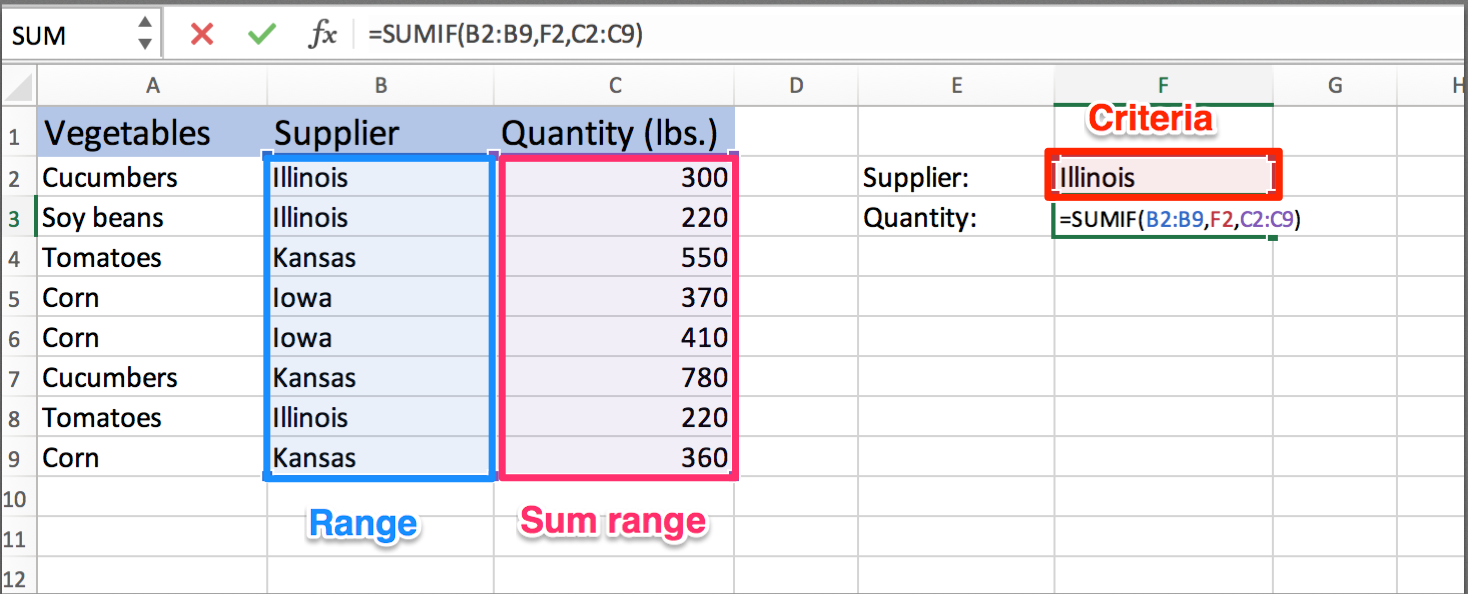Step 4: Press enter

Ta-da! You’re done. Once all the arguments are filled out, all that’s left to do is press enter to get the final quantity. No number-crunching necessary.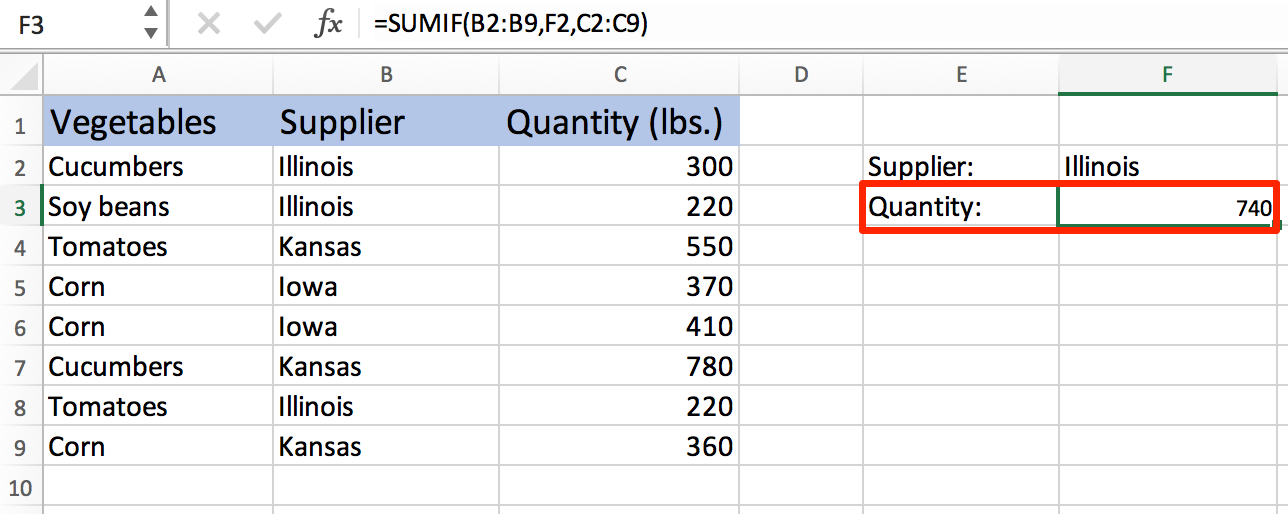If for some reason the final total doesn’t look correct, you can always click on the cell next to “Quantity” and see exactly which cells the data was pulled from.

 Tip: Interested in more Excel tips? Learn how to divide in Excel

Excelling at Excel

It’s as simple as that. Manually crunching numbers is a thing of the past. Excel is a useful tool with hundreds of functions and capabilities that exist to help make your life easier. With some practice, you’ll be a SUMIF expert in no time.

Do you use Microsoft Excel for work? We’d love to hear your feedback. Share your honest thoughts on the tool by writing a review on G2.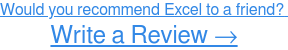Izabelle Hundrev

Izabelle is a Content Marketing Associate who joined G2 in April 2018. After earning a degree in Journalism from the University of Missouri, Izabelle moved back to her hometown of Chicago in pursuit of a career and deep dish pizza. Outside of work, she is passionate about all things pop culture, food, and travel.